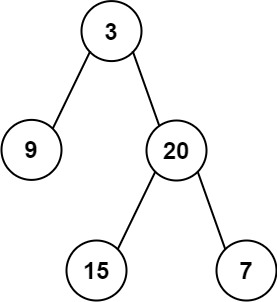0105. 从前序与中序遍历序列构造二叉树

# 0105. 从前序与中序遍历序列构造二叉树#

• 标签：树、数组、哈希表、分治、二叉树
• 难度：中等

## 题目大意 #

• $1 \le preorder.length \le 3000$。
• $inorder.length == preorder.length$。
• $-3000 \le preorder[i], inorder[i] \le 3000$。
• preorderinorder 均无重复元素。
• inorder 均出现在 preorder
• preorder 保证为二叉树的前序遍历序列。
• inorder 保证为二叉树的中序遍历序列。

• 示例 1：1 2  输入: preorder = [3,9,20,15,7], inorder = [9,3,15,20,7] 输出: [3,9,20,null,null,15,7] 
• 示例 2：
 1 2  输入: preorder = [-1], inorder = [-1] 输出: [-1] 

## 解题思路 #

### 思路 1：递归遍历 #

1. 从前序遍历顺序中当前根节点的位置在 postorder
2. 通过在中序遍历中查找上一步根节点对应的位置 inorder[k]，从而将二叉树的左右子树分隔开，并得到左右子树节点的个数。
3. 从上一步得到的左右子树个数将前序遍历结果中的左右子树分开。
4. 构建当前节点，并递归建立左右子树，在左右子树对应位置继续递归遍历并执行上述三步，直到节点为空。

### 思路 1：代码 #

  1 2 3 4 5 6 7 8 9 10 11 12 13  class Solution: def buildTree(self, preorder: List[int], inorder: List[int]) -> TreeNode: def createTree(preorder, inorder, n): if n == 0: return None k = 0 while preorder != inorder[k]: k += 1 node = TreeNode(inorder[k]) node.left = createTree(preorder[1: k+1], inorder[0: k], k) node.right = createTree(preorder[k+1:], inorder[k+1:], n-k-1) return node return createTree(preorder, inorder, len(inorder)) 

### 思路 1：复杂度分析 #

• 时间复杂度：$O(n)$，其中 $n$ 是二叉树的节点数目。
• 空间复杂度：$O(n)$。递归函数需要用到栈空间，栈空间取决于递归深度，最坏情况下递归深度为 $n$，所以空间复杂度为 $O(n)$。